Download Presentation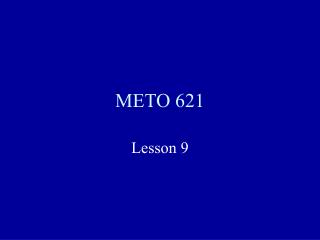METO 621

# METO 621 - PowerPoint PPT Presentation

METO 621. Lesson 9. Solution for Zero Scattering. If there is no scattering, e.g. in the thermal infrared, then the equation becomes. This equation can be easily integrated using an integrating factor e t. Solution for Zero Scattering. Solution for Zero Scattering.I am the owner, or an agent authorized to act on behalf of the owner, of the copyrighted work described.
Download Presentation## METO 621

An Image/Link below is provided (as is) to download presentation

Download Policy: Content on the Website is provided to you AS IS for your information and personal use and may not be sold / licensed / shared on other websites without getting consent from its author.While downloading, if for some reason you are not able to download a presentation, the publisher may have deleted the file from their server.

- - - - - - - - - - - - - - - - - - - - - - - - - - E N D - - - - - - - - - - - - - - - - - - - - - - - - - -
Presentation Transcript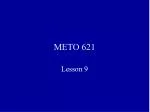### METO 621

Lesson 9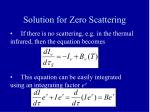Solution for Zero Scattering
• If there is no scattering, e.g. in the thermal infrared, then the equation becomes
• This equation can be easily integrated using an integrating factor et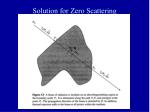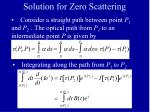Solution for Zero Scattering
• Consider a straight path between point P1 and P2. The optical path from P1to an intermediate point P is given by
• Integrating along the path from P1 to P2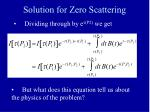Solution for Zero Scattering
• Dividing through by et(P2) we get
• But what does this equation tell us about the physics of the problem?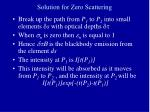Solution for Zero Scattering
• Break up the path from P1 to P2 into small elements ds with optical depths d
• When sn is zero then en is equal to 1
• Hence dtB is the blackbody emission from the element ds
• The intensity at P1 is I[t(P1)]
• This intensity will be absorbed as it moves from P1 to P2 , and the intensity at P2 will be I[t(P1)]exp[-(t(P2)-t(P1)]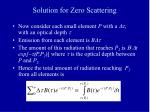Solution for Zero Scattering
• Now consider each small element P with a Dt, with an optical deptht
• Emission from each element is BDt
• The amount of this radiation that reaches P2 is B Dtexp[-t(P,P2)] where t is the optical depth between P and P2
• Hence the total amount of radiation reaching P2from all elements is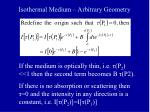Isothermal Medium – Arbitrary Geometry

If the medium is optically thin, i.e. τ(P2) <<1 then the second term becomes B τ(P2).

If there is no absorption or scattering then τ=0 and the intensity in any direction is a constant, i.e. I[τ(P2)]=I[τ(P1)]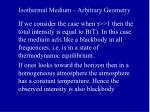Isothermal Medium – Arbitrary Geometry

If we consider the case when τ>>1 then the total intensity is equal to B(T). In this case the medium acts like a blackbody in all frequencies, i.e. is in a state of thermodynamic equilibrium.

If ones looks toward the horizon then in a homogeneous atmosphere the atmosphere has a constant temperature. Hence the observed intensity is also blackbodyZero Scattering in Slab Geometry
• Most common geometry in the theory of radiative transfer is a plane-parallel medium or a slab
• The vertical optical path (optical depth) is given the symbol t as distinct from the slant optical path ts
• Using z as altitude t(z) = ts |cosq|= ts m
• The optical depth is measured along the vertical downward direction, i.e. from the ‘top’ of the mediumHalf-Range Quantities in Slab Geometry
• The half-range intensities are defined by:
• Note that the negative direction is for the downward flux,Half-Range Quantities in Slab Geometry
• The radiative flux is also defined in terms of half-range quantities.Half Range Quantities
• In the limit of no scattering the radiative transfer equations for the half-range intensities becomeFormal Solution in Slab Geometry
• Choose the integrating factor e t/m,, for the first equation, then
• This represents a downward beam so we integrate from the “top” of the atmosphere (t=0) to the bottom (t=t*).Slab Geometry
• For an interior point, t < t* , we integrate from 0 to t. The solution is easily found by replacing t* with t